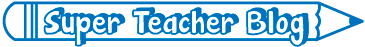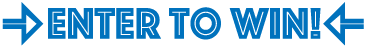# What's New on STW

We add new printable worksheets, games, and teaching tools all the time! This page highlights some of our most recent additions to the site.

496 new files added over the past 365 days.(added April 7, 2021)
Search for simple grammar, mechanics, and spelling mistakes in these ten sentences.
(added April 7, 2021)
Make corrections to the sentences. Look for errors in capitalization, punctuation, and basic sight words.
(added April 7, 2021)
Count by 10s. Then solve four basic addition and subtraction word problems.
(added April 7, 2021)
Read a line plot and answer questions. Multiply 2-digit by 1-digit numbers together. Use addition, subtraction, and multiplication to calculate costs. Solve a word problem involving fractions.
(added April 7, 2021)
THis set includes 1 single-step and 4 multi-step word problems for fourth graders. Multiply fractions by whole numbers, calculate perimeter, and more.
(added March 30, 2021)
Use non-standard units to compare lengths of a guitar and a drum. Use place value blocks to solve subtraction and addition problems. Answer questions about a bar graph. Draw hands on a clock.
(added March 30, 2021)
Find 10 more and 10 less than a given number. Solve a column addition problem (2-digit plus 1-digit). Identify flat surfaces of a cone. Measure a pencil and crayon with non-standard units.
(added March 30, 2021)
Add and using place value blocks. Solve addition and subtraction word problems. Draw hands on an analog clock (nearest hour). Identify flat faces of a cylinder.
(added March 30, 2021)
Draw hands on a clock. Find related math facts. Add using place value blocks. Solve addition/subtraction word problems. Find the sum of three single-digit addends.
(added March 30, 2021)
Color the date on a calendar. Measure a marker with non-standard units. Compare two digit numbers using <, >, and =. Solve basic addition and subtraction word problems.Have you seen the Super Teacher Blog? We post fun, new teaching ideas every month.
Be sure to check it out. Go To STW's Blog
(added March 30, 2021)
Complete a number bond (fact family). Tell how many tens and ones it takes to make 43. Tell time on an analog clock. Measure a glue stick using non-standard units.
(added March 30, 2021)
Tell the time shown on the analog clocks. Tell how many equal parts are in each fraction diagram. Measure a flashlight using nonstandard units. Compare 2-digit numbers.
(added March 30, 2021)
Find the missing numbers (by adding and subtracting one). Determine which 3D shaped have curved surfaces. Answer questions about a pictograph. Tell how many equal parts are shown on circles. Measure a shoe using non-standard units.
(added March 30, 2021)
Here's a picture of Joe Biden that students can color. Below the portrait is an inspirational quotation from Biden.
(added March 25, 2021)
Color only the zebras with the letter Z z on them.
(added March 25, 2021)
Color only the yo-yos with the letter Y y on them.We give away a different prize for teachers each month.
Enter to win on our contest page! Go to STW's Contest Page
(added March 25, 2021)
Color only the dinosaurs with the letter X x on them.
(added March 25, 2021)
Color only the magic wands with the letter W w.
(added March 25, 2021)
There are a dozen flower vases pictured on this worksheet. Color only the ones with the letter V v.
(added March 25, 2021)
There are eleven trucks on this page. Color only the ones with the letter T t on them.
(added March 25, 2021)
There are 12 seashells pictures. Color only the ones with the letter S s on them.
(added March 25, 2021)
3-2-1 blast off! Color only the rocket ships with the letter R r on them.
¿Habla español?
Many of our worksheets have been translated into Spanish! Click thebutton below worksheet descriptions to view our Spanish translations. Learn more.
(added March 25, 2021)
Color the crowns with Q q on them. This version has lowercase q with a sharp pointed tail.
(added March 25, 2021)
Color the crowns with Q q. This version has lowercase q with a straight line tail.
(added March 25, 2021)
Color only the crowns with the letter Q q on them. This version has the lowercase q with a curl on the tail.
(added March 25, 2021)
Color only the pigs with the letter P p on them.
(added March 25, 2021)
Color only the owls with the letter O o on them.
(added March 25, 2021)
Color only the nuts with the letter N n on them.
(added March 25, 2021)
Color only the light bulbs with the letter L l.
(added March 25, 2021)
Color only the mugs with the letter M m on them.
(added March 22, 2021)
Count by twos starting with 2 (2, 12, 22, 32, 42...), practice repeated addition, as well as basic addition and subtraction.
(added March 22, 2021)
On these worksheets, students will count by tens starting with (8, 18, 28, 38...), complete a part-part-whole diagram, complete a fact family, and use place value blocks to find a number.
(added March 19, 2021)
Read each sentence carefully and look for errors. Correct the mistakes in spelling, punctuation, grammar, and capitalization.
(added March 19, 2021)
Find and correct the errors in these twelve Christmas-themed sentences.
(added March 18, 2021)
Circle the correct type of angle and find the unknown measurement. There are 12 problems on this worksheet.
(added March 18, 2021)
This page has information about complementary, supplementary, and vertical angles, with examples. At the bottom, students identify the angle type and calculate the unknown measurements.
(added March 18, 2021)
Identify complementary, supplementary, and vertical angles. Calculate the number of degrees in each unknown angle.
(added March 17, 2021)
Skills on this set of worksheets includes: reading and interpreting a line plot; solving a basic multiplication word problem; solving a basic division problem; and completing a multi-step problem involving multiplication and addition.
(added March 17, 2021)
This file includes a multi-step problem that requires both subtraction and multiplication. There is also a problem where students must draw an array to multiply.
(added March 17, 2021)
In these single-step and multi-step problems, your students will review repeated addition (basic multiplication), as well as addition and subtraction.
(added March 16, 2021)
Color only the eggs that have the letter E e on them.
(added March 16, 2021)
Color only the cupcakes with the letter C c on them.
(added March 16, 2021)
Color only the hearts that have the letter H h on them.
(added March 16, 2021)
Color only the ice cream cones with the letter I i printed on them.
(added March 16, 2021)
Color only the keys that have the letter K k on them.
(added March 16, 2021)
Color only the jars with the letter J j in them.
(added March 16, 2021)
Solve the 2-digit by 1-digit multiplication problems. Then use the answers to decode the solution to a silly riddle. Why did the cow do jumping jacks? She wanted to make a milkshake.
(added March 16, 2021)
In this week's worksheet set, students will practice counting by 10s (6, 16, 26, 36...), find "one more" and "one less" than a given number, write the times shown on the analog clocks (11:00 and 1:00), and illustrate a math fact family.
(added March 16, 2021)
On these Math Buzz worksheets, your first graders will measure a whale using non-standard units, identify solids (cones, cylinders, cubes), subtract, and identify shapes that have equal parts.
(added March 16, 2021)
In this set, pupils will identify cubes, spheres, and cylinders. They will also complete an addition bar model, use non-standard units to measure a lizard, and compare two digit numbers (using <, >, and =).
Please Log In to Super Teacher Worksheets
Username:
Password:
Can't remember your password?
My Account
Site Information
Useful LinksNot a Member?
For complete access to thousands of printable lessons click the button or the link below.
© 2021 Super Teacher Worksheets

PDF with answer key:

PDF no answer key:

Common Core Alignment
Preview